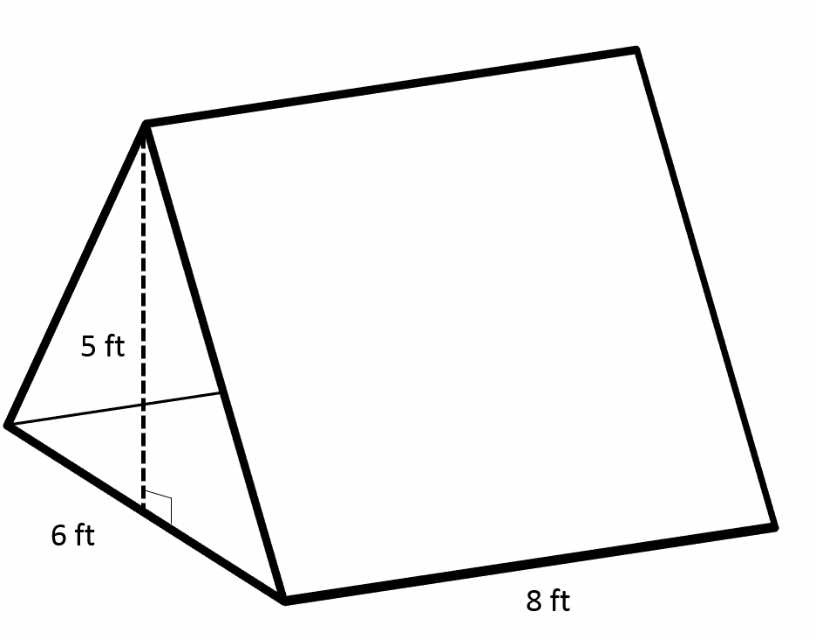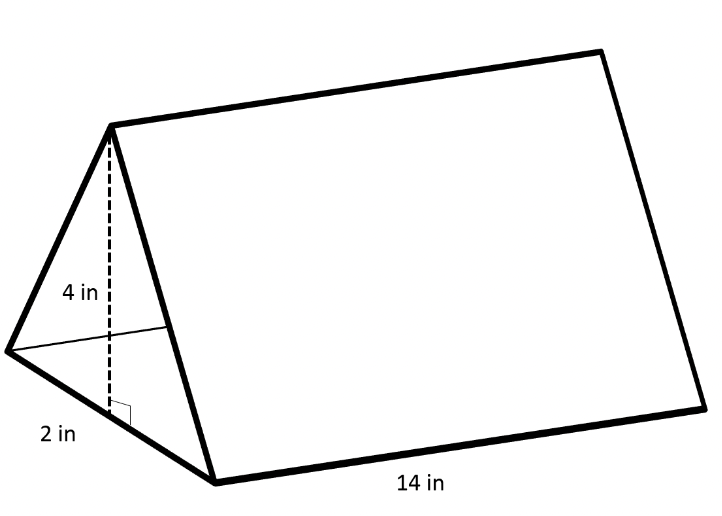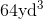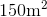# ACT Mathematics: Solid Geometry Quiz

This is an MCQ-based quiz on the topic of ACT Mathematics: Solid Geometry Quiz.

This set of MCQs helps you brush up on the important mathematics topic and prepare you to dive into skill practice and expand your knowledge to tackle the question and answer it carefully.

Start Quiz

The tent shown below is in the shape of a triangular prism. What is the volume of this tent in cubic feet?360

120

80

240

Susan bought a chocolate bar that came in a container shaped like a triangular prism shown below. If the container is completely filled with chocolate, in cubic inches, what volume of chocolate did Susan buy?78

56

112

90

Troy's company manufactures dice that are shaped like cubes and have side lengths of 2cm. If the plastic needed to make the dice costs \$0.10 per cubic centimeter, how much does it cost Troy to make one die?

0.4

0.6

0.8

1.6

The height of a box is twice its width and half its length. If the volume of the box is, what is the length of the box?

2

4

6

8

A cube has a surface area of. What is its volume?

125

150

100

200

Matt baked a rectangular cake for his mom's birthday. The cake was 15 inches long, 12 inches wide, and 4 inches high. If he cuts the cake into pieces that are 3 inches long, 2 inches wide, and 4 inches high, how many pieces of cake can he cut?

30

20

40

10

If we have a regular (the triangles are equilateral) triangular prism of volume 32in3 and the side length of the triangle on either face is 3in, what is the length of the prism? Write your answer in terms of a decimal rounded to the nearest hundredth.

12.32

14.23

15.46

18.90

Roberto has a swimming pool that is in the shape of a rectangular prism. His swimming pool is 25 meters wide, 10 meters long, and 3 meters deep. He needs to fill up the pool for summer, and his hose fills at a rate of 5 cubic meters per hour. How many hours will it take for Roberto to fill up the swimming pool?

200

150

40

90

Quiz/Test Summary
Title: ACT Mathematics: Solid Geometry Quiz
Questions: 8
Contributed by: